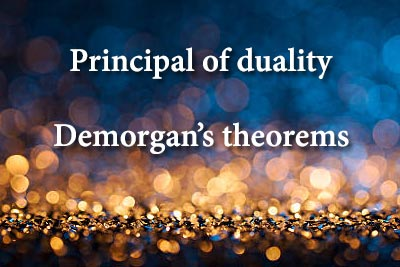Principal of duality and Demorgan's theorems - Digital Electronics
08-03-2019    119 times### Principal of duality

One expression can be obtain from another expression by replacing the every 1 with 0, every 0 with 1, every (+) with (.), every (.) with (+). Any pair of expression satisfying this property is called dual expression. This characteristic of Boolean algebra is called the principle of duality.

### Demorgan’s Theorems

Two important theorems are proposed by DeMorgan. This two theorems have very important role in Boolean expression.

Theorem I: The Complement of a product is equal to the sum of the complements.

A.B = A + B

Theorem II: The Complement of a sum is equal to the product of the complements.

A + B = A . B

### Proof for DeMorgan’s theorems

 A B A B A+B A.B A+B A . B A . B A + B 0 0 1 1 0 0 1 1 1 1 0 1 1 0 1 0 0 0 1 1 1 0 0 1 1 0 0 0 1 1 1 1 0 0 1 1 0 0 0 0Query About the post